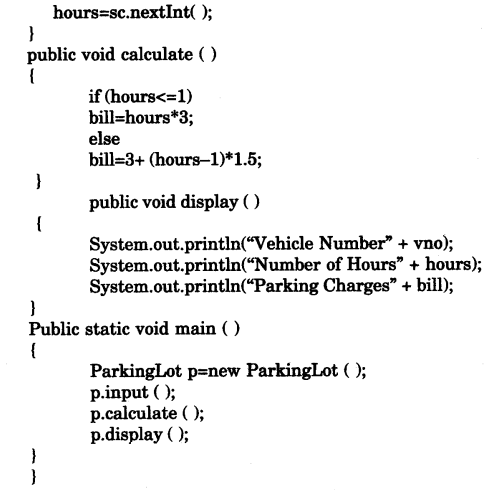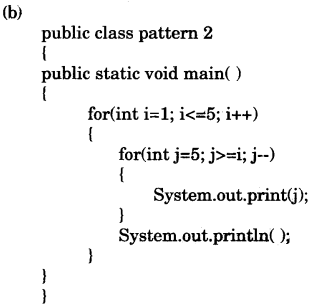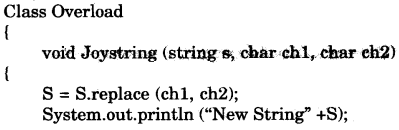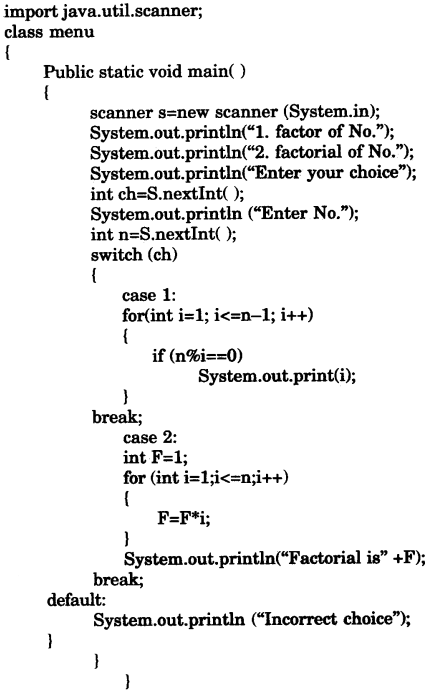## ICSE Computer Applications Previous Year Question Paper 2015 Solved for Class 10

ICSE Paper 2015
COMPUTER APPLICATIONS

(Two Hours)
Answers to this Paper must be written on the paper provided separately.
You will not be allowed to write during the first 15 minutes.
This time is to be spent in reading the question paper.
The time given at the head of this Paper is the time allowed for writing the answers.
This paper is divided into two Sections.
Attempt all questions from Section A and any four questions from Section B.
The intended marks for questions or parts of questions are given in brackets [ ].

Section ‘A’ (40 Marks)

(Attempt all questions)

Question 1:
(a) What are the default values of the primitive data type int and float ? 
(b) Name any two OOP’s principles. 
(c) What are identifiers ? 
(d) Identify the literals listed below: 
(i) 0.5 (ii) ‘A’ (iii) false (iv) “a”
(e) Name the wrapper classes of char type and boolean type. 

(a) Default values of:
int 0
float 0.0

(b) Two OOP’s principles :
(i) Abstraction (ii) Inheritance.

(c) Identifiers: Identifiers are the symbolic names given to variables, objects, classes etc.

(d) (i) 0.5—double type literal. (ii) ‘A’—char type literal.
(iii) false—boolean type literal. (iv) “a”—string type literal.

Question 2:
(a) Evaluate the value of a. if value of p = 5, q = 19  
int n = (q – p) > (p – q) ? (q – p) : (p – q);

(b) Arrange the following primitive-data types in an ascending order of their size:
(i) char (ii) byte (iii) double (iv) int 

(c) What is the value stored in variable res given below :
double res = Math.pow (“345”.indexOf(‘5’), 3); 

(d) Name the two types of constructors 

(e) What are the values of a and b after the following function is executed, if the values passed are 30 and 50: 
void paws(int a, int b)
{
a=a + b;
b=a – b;
a=a – b;
System.out.println (a+ “,” +b);
}

(a) 14.

(b) byte, char, int, double.

(c) res =8

(d) Two types of constructor :
(i) default constructor
(ii) parameterized constructor.

(e) The value of a will be 50 and
The value of b will be 30.

Question 3:
(a) State the data type and value of y after the following is executed:
char x=7;
y-Character.isLetter(x); 

(b) What is the function of catch block in exception handling ? Where does it appear in a program ? 

(c) State the output when the following program segment is executed:
String a =“Smartphone”, b=“Graphic Art”;
String h=a.substring(2, 5);
String k=b.substring(8).toUpperCase();
System.out.println(h);
System.out.println(k.equalsIgnoreCase(h)); 

(d) The access specifier that gives the most accessibility is ………… and the least accessibility is ………… . 

(e) (i) Name the mathematical function which is used to find sine of an angle given in radians.
(ii) Name a string function which removes the blank spaces provided in the prefix and suffix of a string. 

(f) (i) What will this code print ?
int arr[]=new int;
System.out.println(arr);
(i) 0
(ii) value stored in arr
(iii) 0000
(iv) garbage value
(ii) Name the keyword which is used- to resolve the conflict between method parameter and instance variables/fields. 

(g) State the package that contains the class :
(ii) Scanner 

(h) Write the output of the following program code: 
char ch ;
int x=97;
do
{
ch=(char) x;
System.out.print(ch + “ ” );
if(x%10 == 0)
break;
++x;
}while(x<=100);

(i) Write the Java expressions for $$\frac { { a }^{ 2 }+{ b }^{ 2 } }{ 2ab }$$ 
(ii) If int y = 10 then find int z = (++y * (y++ +5)); 

(a) y = false.
data type of y is boolean.

(b) Catch block is used as exception handler in exception handling. We can put the code to deal with the execution that might arise, in this block. Catch block must appear just below the tiy block.

(c) Output:
art
true.

(d) The access specifier that gives the most accessibility is public and the least accessibility is private.

(e) (i) Math.sin() (ii) trim ()

(f) (i) garbage value. (ii) ‘this’ keyword.

(g) (i) java.io package. (ii) java.util package.

(h) output: a b c d

(i) R = a*a + b*b/2*a*b;

(j) z = (11 * (11 + 5))
= 11 * 16
= 176

SECTION B (60 Marks)

Attempt any four questions from this Section.
The answers in this Section should consist of the Programs in either Blue J environment or any program environment with Java as the base.
Each program should be written using Variable descriptions /Mnemonic Codes so that the logic of the program is clearly depicted.
Flow-Charts and Algorithms are not required.

Question 4:
Define a class named ParkingLot with the following description :
Instance variables/data members:
int vno — To store the vehicle number
int hours — To store the number of hours the vehicle is parked in the parking lot
double bill — Tb store the bill amount
Member methods:
void input( ) — To input and store the vno and hours.
void calculate( ) — To compute the parking charge at the rate of Rs.3 for the first hours or part thereof, and Rs. 1.50 for each additional hour or part thereof.
void display ( ) — To display the detail
Write a main method to create an object of the class and call the above methods.Question 5:Question 6:
Write a program to input and store roll numbers, names and marks in 3 subjects of n number students in five single dimensional array and display the remark based on average marks as given below : (The maximum marks in the subject are 100)Question 7:
Design a class to overload a function Joystring( ) as follows :
(i) void Joystring (String s, char ch1 char ch2) with one string argument and two character arguments that replaces the character argument ch1 with the character argument ch2 in the given string s and prints the new string.
Example:
Input value of s = “TECHNALAGY”
ch1=‘A’,
ch2=‘O’
Output: TECHNOLOGY
(ii) void Joystring (String s) with one string argument that prints the position of the first space and the last space of the given string s.
Example:
Input value of = “Cloud computing means Internet based computing”
Output: First index : 5
Last index : 36
(iii) void Joystring (String s1, String s2) with two string arguments that combines the two string with a space between them and prints the resultant string. Example :
Input value of s1 =“COMMON WEALTH”
Input value of s2 =“GAMES”
Output: COMMON WEALTH GAMES
(use library functions)Question 8:
Write a program to input twenty names in an array. Arrange these names in descending order of alphabets, using the bubble sort technique.Question 9:
Using the switch statement, write a menu driven program to:
(i) To find and display all the factors of a number input by the user (including 1 and excluding number itself).
Example:
Sample Input: n=15
Sample Output: 1, 3, 5.
(ii) To find and display the factorial of a number input by the user (the factorial of a non-negative integer n, denoted by n\ is the product of all integers less than or equal to n.
Example:
Sample Input: n=5
Sample Output: 5! = 1×2×3×4×5 = 120.
For an incorrect choice, an appropriate error message should be displayed.